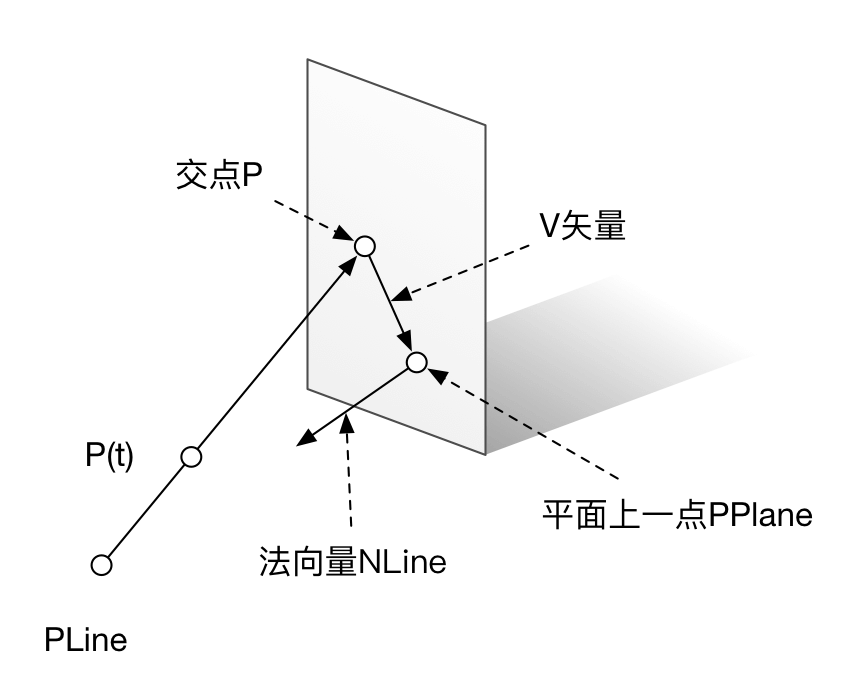# 如何写一个球面Ray tracing程序(1)

## 直线与平面的交点¶### 用参数方程求交点¶

$$dot(P_{Line}+t \times N_{Line}-P_{Plane}, N_{Plane})=0$$

dot product是满足分配律的，于是有 $$dot(P_{Line}-P_{Plane}, N_{Plane}) + dot( t \times N_{Line}, N{Plane})=0$$

$$t= - \frac{dot(P_{Line}-P_{Plane}, N_{Plane})}{dot( N_{Line}, N{Plane})}$$

$$t=\frac{dot(P_{Plane}-P_{Line}, N_{Plane})}{dot( N_{Line}, N{Plane})}$$

In :
import numpy as np
def lineCrossPlane(PLine, NLine,PPlane,NPlane):
'''
直线以起点PLine和方向向量NLine表示
平面以平面上一点PPlane和法向量NPlane表示
求其交点P
直线有多组，平面仅一个
'''
tup=np.dot((PPlane-PLine),NPlane)
tdown=np.dot(NLine,NPlane)
t=tup/tdown
t=np.expand_dims(t,axis=1) # 为了满足与t与NLine的乘法, 需要将t扩展一维
P=PLine+t*NLine
return P

In :
# 测试结果:
if __name__=="__main__":
pline=np.array([[0,0,0],[0,1,2]])
nline=np.array([[1,0,0],[1,0,0]])
pplane=np.array([5,0,0])
nplane=np.array([1,0,0])
print(lineCrossPlane(pline,nline,pplane,nplane))

[[ 5.  0.  0.]
[ 5.  1.  2.]]

In [ ]: